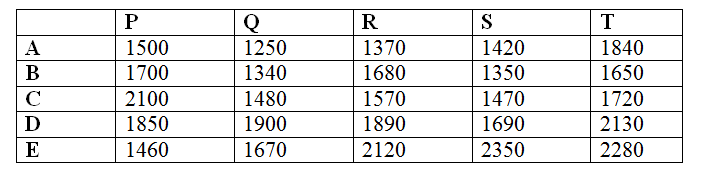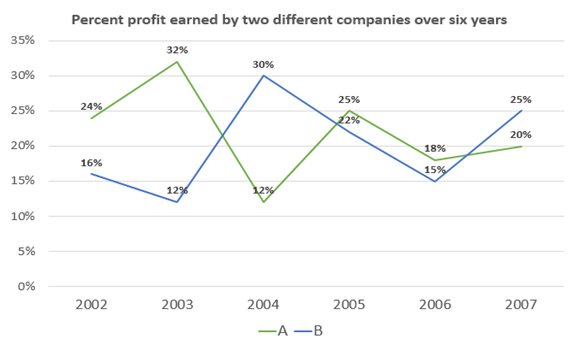# Quantitative Aptitude Questions (Data Interpretation) for IBPS RRB Assistant Prelims 2018 Day-169

Dear Readers, IBPS RRB is conducting Online Examination for the recruitment of clerical cadre To enrich your preparation here we have providing new series of Data Interpretation – Quantitative Aptitude Questions. Candidates those who are appearing in IBPS RRB Assistant Prelims Exams can practice these Quantitative Aptitude average questions daily and make your preparation effective.

[WpProQuiz 3262]

Click “Start Quiz” to attend these Questions and view Solutions

Directions (Q. 1 – 5) Study the following information carefully and answer the given questions:

Following table shows the sales of different types of items (A, B, C, D, E) in different shops (P, Q, R, S, T) in a certain week.1) Find the ratio between the total number of sales of type C to that of type E in all the given shops together?

a) 234 : 117

b) 315 : 429

c) 572 : 623

d) 417 : 494

e) None of these

2) Find the difference between the total sales in shop P to that of shop R of all the given types of items together?

a) 50

b) 20

c) 30

d) 40

e) None of these

3) Find the average number of sales of type A items in all the given shops together?

a) 1338

b) 1476

c) 1274

d) 1152

e) None of these

4) Total number of type B items sold by shop Q, S and T together is approximately what percentage of total number of type D items sold by shop P, R and S together?

a) 60 %

b) 70 %

c) 80 %

d) 95 %

e) None of these

5) Total number of type C and D items sold by shop T is approximately what percentage more/less than the total number of type A and D items sold by shop Q?

a) 22 % more

b) 15 % less

c) 8 % less

d) 12 % more

e) 5 % more

Directions (Q. 6 – 10) Study the following information carefully and answer the given questions:6) Sum of the percent profit earned by company A in all the years together is what percent of the sum of the percent profit earned by company B in all the years together?

a) 120 %

b) 75 %

c) 90 %

d) 110 %

e) 100 %

7) If the expenditures of companies A and B in 2004 are same, then find the respective ratio of their incomes?

a) 45 : 54

b) 56 : 65

c) 65 : 56

d) 54 : 45

e) None of these

8) If the incomes of company A and B in 2006 were Rs. 177000 and Rs. 201250 respectively, then the expenditure of A is approximately what percent less than the expenditure of B?

a) 40%

b) 25%

c) 15%

d) 55%

e) 5%

9) If the expenditure of B for all the years is Rs. 65000, then find the sum of incomes in all the years?

a) Rs.498000

b) Rs.468000

c) Rs.488000

d) Rs.478000

e) None of these

10) Find the respective ratio of the incomes of A in 2007 and B in 2005, if their respective expenditures are Rs. 78000 and Rs. 95000?

a) 853 : 1097

b) 857 : 1098

c) 932 : 1157

d) 936 : 1159

e) None of these

Direction (1-5)

Explanation:

The total number of sales of type C in all the given shops together

= > 2100 + 1480 + 1570 + 1470 + 1720 = 8340

The total number of sales of type E in all the given shops together

= > 1460 + 1670 + 2120 + 2350 + 2280 = 9880

Required ratio = 8340 : 9880 = 417 : 494

Explanation:

The total sales in shop P of all the given types of items together

= > 1500 + 1700 + 2100 + 1850 + 1460 = 8610

The total sales in shop R of all the given types of items together

= > 1370 + 1680 + 1570 + 1890 + 2120 = 8630

Required difference = 8630 – 8610 = 20

Explanation:

The total number of sales of type A items in all the given shops together

= > 1500 + 1250 + 1370 + 1420 + 1840 = 7380

Required average = 7380/5 = 1476

Explanation:

Total number of type B items sold by shop Q, S and T together

= > 1340 + 1350 + 1650 = 4340

Total number of type D items sold by shop P, R and S together

= > 1850 + 1890 + 1690 = 5430

Required % = (4340/5430)*100 = 80 %

Explanation:

Total number of type C and D items sold by shop T

= > 1720 + 2130 = 3850

Total number of type A and D items sold by shop Q

= > 1250 + 1900 = 3150

Required % = [(3850 – 3150)/3150]*100 = 22 % more

Direction (6-10)

Explanation:

Sum of the percent profit earned by company A in all the years together

= > 24 + 32 + 12 + 25 + 18 + 20 = 131

Sum of the percent profit earned by company B in all the years together

= > 16 + 12 + 30 + 22 + 15 + 25 = 120

Required percentage = (131/120)*100 = 110 %

Explanation:

Let the expenditures of company A and B in 2004 be Rs. x,

Income of A in 2004 = x * 112/100 = 28x/25

Income of B in 2004 = x * 130/100 = 13x/10

Required ratio = 28x/25 : 13x/10 = 56 : 65

Explanation:

Expenditure of A in 2006

= > [100/(100 + 18)]*177000 = Rs. 150000

Expenditure of B in 2006

= > [100/(100 + 15)]*201250 = Rs. 175000

Required percentage

= > [(175000 – 150000)/175000]*100 = 15 %

Explanation:

Sum of the incomes in all the years

= > 65000*(116/100) + 65000*(112/100) + 65000*(130/100) + 65000*(122/100) + 65000*(115/100) + 65000*(125/100)

= > 75400 + 72800 + 84500 + 79300 + 74750 + 81250

= > Rs. 468000

Explanation:

Income of A in 2007 = 78000*(120/100) = Rs. 93600

Income of B in 2005 = 95000*(122/100) = Rs. 115900

Required ratio = 93600 : 115900 = 936 : 1159

Daily Practice Test Schedule | Good Luck

 Topic Daily Publishing Time Daily News Papers & Editorials 8.00 AM Current Affairs Quiz 9.00 AM Quantitative Aptitude “20-20” 11.00 AM Vocabulary (Based on The Hindu) 12.00 PM General Awareness “20-20” 1.00 PM English Language “20-20” 2.00 PM Reasoning Puzzles & Seating 4.00 PM Daily Current Affairs Updates 5.00 PM Data Interpretation / Application Sums (Topic Wise) 6.00 PM Reasoning Ability “20-20” 7.00 PM English Language (New Pattern Questions) 8.00 PM